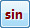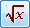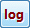ESB Calculators and Unit Conversion Utilities
Prefix Functions and Precedence

 Home ESBCalc Pro Suite ESBCalc Pro ESBUnitConv Pro ESBProgCalc Pro ESBFinCalc Pro ESBDateCalc Pro ESBCalc (free) ESBUnitConv (free) Prefix Fns / Precedence Purchase License Details Rec. Products Link to Us

Prefix Functions

Traditionally, in non-RPN Calculators, functions were entered after the expression - so when in Mathematics you would write "sin 2.5" on a calculator you would press 2.5 then press.

Thus software calculators started out this way as well.

However many modern physical calculators allow you to enter your formulae the same way you write it mathematically. So if the function occurs first then you press it first. So in the above you pressthen 2.5. We refer to this as having Prefix Functions On.

Prefix Functions can be turned off - see Optons.

Examples

Sqrt 2

Prefix On:then 2

Prefix Off:  2 thenlog 4.5

Prefix On:then 4.5

Prefix Off:  4.5 then52

Prefix On and Prefix Off:  5 then(as this is not a function that is written in Prefix mode)

72.5

Prefix On and Prefix Off:  7 thenthen 2.5 (as this is not a function that is written in Prefix mode)

Precedence

According to standard arithmetic, multiplication and division have precedence over addition and subtraction - this means they are done first.

So 2 + 3 x 4 means that the calculator works out the multiplication first, then the addition. So this evaluates to 14, NOT 20!

If you want the addition to evaluate first, then you need to use brackets:

( 2 + 3 ) x 4 = 20

To implement this, the calculator maintains a stack of values (operands) and a stack of operators.

Normal Precedence can be turned off in the Options.